# 碰撞检测

Note

## AABB - AABB 碰撞

AABB代表的是轴对齐碰撞箱(Axis-aligned Bounding Box)，碰撞箱是指与场景基础坐标轴（2D中的是x和y轴）对齐的长方形的碰撞外形。与坐标轴对齐意味着这个长方形没有经过旋转并且它的边线和场景中基础坐标轴平行（例如，左右边线和y轴平行）。这些碰撞箱总是和场景的坐标轴平行，这使得所有的计算都变得更简单。下边是我们用一个AABB包裹一个球对象（物体）：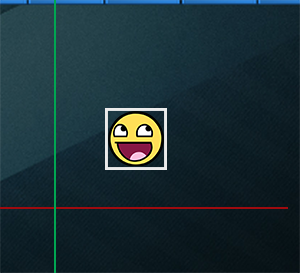Breakout中几乎所有的物体都是基于长方形的物体，因此很理所应当地使用轴对齐碰撞箱来进行碰撞检测。这就是我们接下来要做的。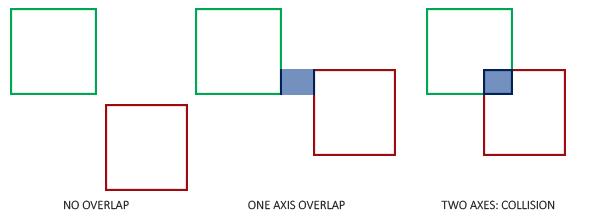GLboolean CheckCollision(GameObject &one, GameObject &two) // AABB - AABB collision
{
// x轴方向碰撞？
bool collisionX = one.Position.x + one.Size.x >= two.Position.x &&
two.Position.x + two.Size.x >= one.Position.x;
// y轴方向碰撞？
bool collisionY = one.Position.y + one.Size.y >= two.Position.y &&
two.Position.y + two.Size.y >= one.Position.y;
// 只有两个轴向都有碰撞时才碰撞
return collisionX && collisionY;
}


class Game
{
public:
[...]
void DoCollisions();
};


void Game::DoCollisions()
{
for (GameObject &box : this->Levels[this->Level].Bricks)
{
if (!box.Destroyed)
{
if (CheckCollision(*Ball, box))
{
if (!box.IsSolid)
box.Destroyed = GL_TRUE;
}
}
}
}


void Game::Update(GLfloat dt)
{
// 更新对象
Ball->Move(dt, this->Width);
// 检测碰撞
this->DoCollisions();
}


## AABB - 圆碰撞检测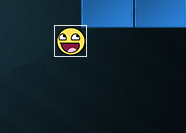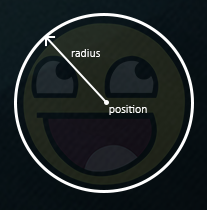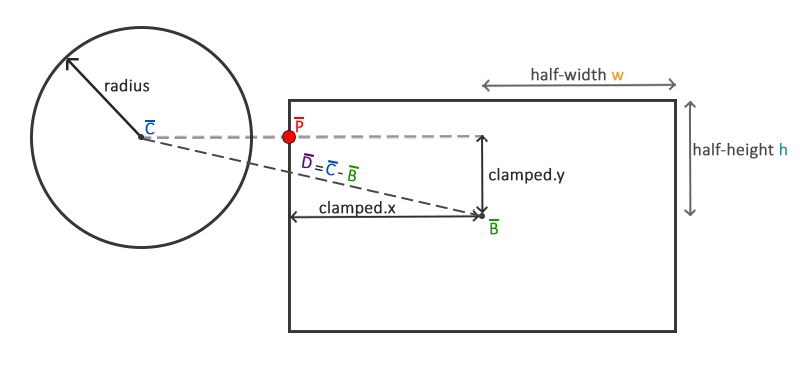Important

float clamp(float value, float min, float max) {
return std::max(min, std::min(max, value));
}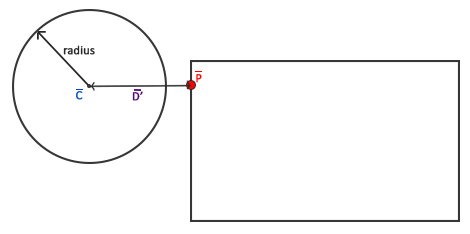GLboolean CheckCollision(BallObject &one, GameObject &two) // AABB - Circle collision
{
// 获取圆的中心
glm::vec2 center(one.Position + one.Radius);
// 计算AABB的信息（中心、半边长）
glm::vec2 aabb_half_extents(two.Size.x / 2, two.Size.y / 2);
glm::vec2 aabb_center(
two.Position.x + aabb_half_extents.x,
two.Position.y + aabb_half_extents.y
);
// 获取两个中心的差矢量
glm::vec2 difference = center - aabb_center;
glm::vec2 clamped = glm::clamp(difference, -aabb_half_extents, aabb_half_extents);
// AABB_center加上clamped这样就得到了碰撞箱上距离圆最近的点closest
glm::vec2 closest = aabb_center + clamped;
// 获得圆心center和最近点closest的矢量并判断是否 length <= radius
difference = closest - center;
return glm::length(difference) < one.Radius;
}